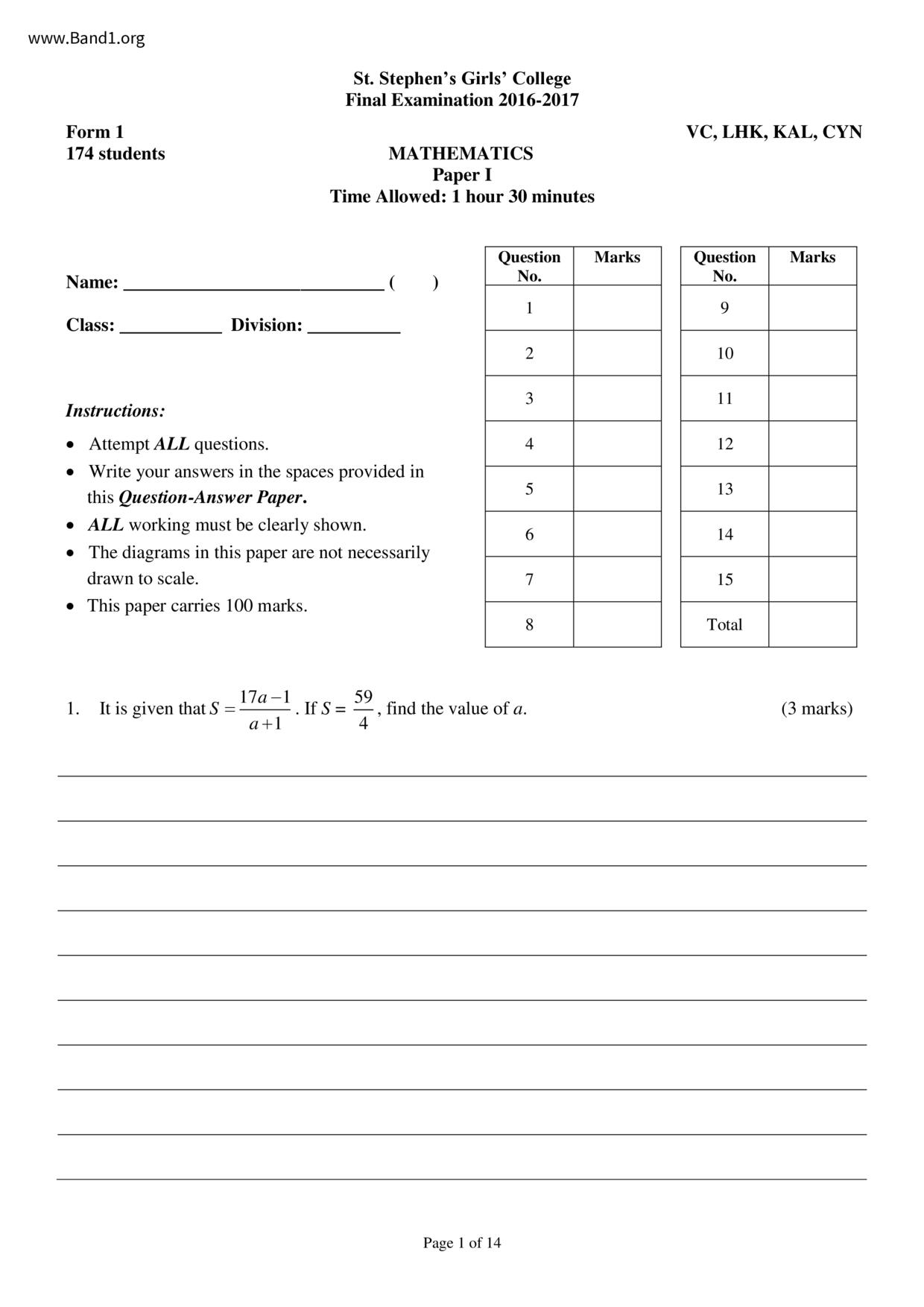# 中一 數學試卷 (F1 Maths Past Paper)

6935

pdf

14

-Ma-P1-16-17-fy

▼ 圖片只作預覽, 如欲下載整份卷, 請按「免費成為會員」 ▼▲ 圖片只作預覽, 如欲下載整份卷, 請按「免費成為會員」 ▲

## 中一數學試卷 PDF 下載

174 students
1. It is given that S =
St. Stephen's Girls' College
Final Examination 2016-2017
Instructions:
• Attempt ALL questions.
• ALL working must be clearly shown.
• The diagrams in this paper are not necessarily
drawn to scale.
• This paper carries 100 marks.
MATHEMATICS
Time Allowed: 1 hour 30 minutes
Page 1 of 14
find the value of a.
VC, LHK, KAL, CYN
Question Marks
F.1 Mathematics Paper I Final Examination 2016-2017
11. In the figure, EGHD, AGB, AHC and BCF are straight lines. ED // BF.
(a) Express ZABC in terms of f.
(b) Find f.
(c) Determine if AB is parallel to DC, and give reasons. (4 marks)
Page 10 of 14
F.1 Mathematics Paper I Final Examination 2016-2017
12. In the figure, U is a point on PQ and PQ // RS.
(a) Express x in terms of a.
(b) If a = 65°, find x.
Page 11 of 14
F.1 Mathematics Paper I Final Examination 2016-2017
13. In the figure, AOB is a straight line and AB LOC. It is given that AC = BC.
(a) Prove that AAOC ABOC.
(b) Find the value of x.
(c) Find the area of AABC.
Page 12 of 14
F.1 Mathematics Paper I Final Examination 2016-2017
14. In the figure, PQ // RS and ZSQP = ZRPS.
(a) Prove that APQS ~ ASPR.
(b) Find the perimeter of the quadrilateral PQSR. (4 marks)
Page 13 of 14
F.1 Mathematics Paper I Final Examination 2016-2017
15. The stem-and-leaf diagram below shows the ages of the employees of a company.
Stem(tens) Leaf(units)
(a) If the difference between the age of the oldest employee and the age of the youngest employee is
less than 35, write down all the possible values of a.
(b) Find the percentage of employees with ages 40 or above.
(c) After 5 years, the two oldest employees will leave the company and the company will not recruit
new employees. Will the percentage of employees with ages 40 or above be greater than the
* End of Paper *
Page 14 of 14
F.1 Mathematics Paper I Final Examination 2016-2017
2. Solve the equation- - x = 1.
3. There are 20 questions in a game. 5 marks will be given for a correct answer. Otherwise, 3 marks will
be deducted.
(a) Peter got x answers correct. Express the marks of Peter in terms of x.
(b) Is it possible for a student to get 0 mark in the game? Explain your answer.
Page 2 of 14
F.1 Mathematics Paper I Final Examination 2016-2017
4. The pie chart below shows the favourite sports of the students in S1A.
It is known that 8 students in S1A choose 'Swimming' as their favourite sport.
(a) Find the total number of students in S1A.
(b) If 30% of students in S1A choose 'Basketball' as their favourite sport, find
the number of students in S1A who choose 'Badminton' as their favourite sport. (3 marks)
Page 3 of 14
F.1 Mathematics Paper 1 Final Examination 2016-2017
5. A(-5, -2), B(3, -1), C(6, 4) and D(0, 6) represent the vertices of a quadrilateral ABCD. Let O be the
(a) Draw the quadrilateral ABCD in a rectangular coordinate plane.
(b) Find the area of ABCD.
(c) The coordinates of a point P are (5, 0).
(i) Mark P in the coordinate plane in (a).
(ii) Hence, determine whether O and P lie inside or outside ABCD.
Page 4 of 14
F.1 Mathematics Paper I Final Examination 2016-2017
6. Consider two points A(3, 5) and B(3,-2). AB cuts the x-axis at C.
(a) Write down the coordinates of C.
(b) K is a point on the x-axis such that the area of A KAB is 14 sq. units. Find the two possible
coordinates of K.
Page 5 of 14
F.1 Mathematics Paper I Final Examination 2016-2017
The figure shows a container in the shape of a prism. The shaded area is the lid of the container. It is
given that the total surface area (including the lid) of the container is 588 cm².
(a) Find the value of x.
(b) Find the capacity of the container.
(c) If the container contains 54% of its capacity of water, find the depth of water if the container is
placed upright as shown.
Page 6 of 14
F.1 Mathematics Paper I Final Examination 2016-2017
Page 7 of 14
F.1 Mathematics Paper I Final Examination 2016-2017
8. In the figure, the shaded region is the base of a metal prism.
(a) Find the area of the shaded region.
(b) It is given that the height of the prism is 12 cm. If the prism is
melted and recast into another prism with height 10 cm, find
base area of the new prism.
9. The figure shows a rectangle R.
(a) Draw the image K after R is translated 4 units to the right.
(b) Draw the image K' after K is rotated clockwise about point P through 270°.
(c) Draw the image R' after R is rotated clockwise about point P through 270°.
(d) If R' undergoes a transformation to K', describe the transformation.
Page 8 of 14
F.1 Mathematics Paper I Final Examination 2016-2017
10. In the figure, point Q is the image of point P after P is rotated anticlockwise about the origin O
through 270°.
(a) Write down the coordinates of P.
(b) Q is translated 18 units upwards and then 2 units to the right to a point R.
(i) Find the coordinates of R.
(ii) If P undergoes a transformation to R, describe the transformation.
(iii) Do P, O and R lie on the same straight line?
Page 9 of 14